## A wheel has a rotational inertia of 16 kgm2. Over an interval of 2.0 s its angular velocity increases from 7.0 rad/s to 9.0 rad/s. What is t

Question

A wheel has a rotational inertia of 16 kgm2. Over an interval of 2.0 s its angular velocity increases from 7.0 rad/s to 9.0 rad/s. What is the average power done by the torque

in progress 0
6 months 2021-08-15T13:48:35+00:00 1 Answers 4 views 0

## 128.61 Watts

Explanation:

Average power done by the torque is expressed as the ratio of the workdone by the toque to time.

Power = Workdone by torque/time

Workdone by the torque =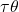=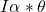I is the rotational inertia = 16kgm²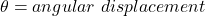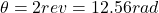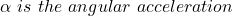To get the angular acceleration, we will use the formula;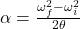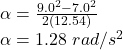Workdone by the torque = 16 * 1.28 * 12.56

Workdone by the torque = 257.23 Joules

Average power done by the torque = Workdone by torque/time

=  257.23/2.0

= 128.61 Watts